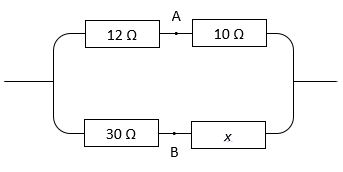# Short Circuit?This circuit, consisting of four resistors, is connected to a voltage source. The value of the resistance marked $x$ is unknown.

When we accidentally drop a screwdriver across the wires, thus connecting points A and B directly, there is no noticeable change in the current that flows through the circuit.

Calculate the resistance $x$, in ohms.

×